Next: Converting (SSP) to (SP) Up: Subset-Sum Problems and NP-Completeness Previous: The Satisfiability Problem   Contents

## Converting (SP) to (SSP)

We want to construct numbersand a target numberso that there is a subset adding up toif and only if there is an assignment for (SP) which makes all the formulas true. Using this construction allows us to use an algorithm for (SSP) to solve (SP). It implies that solving (SSP) is at least as hard as solving (SP).

We will illustrate the construction using the example in Figure 1. We will have numbers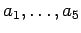corresponding to the logic variables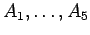with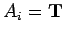corresponding tobeing included in the subset. We will also need additional,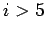for technical reasons.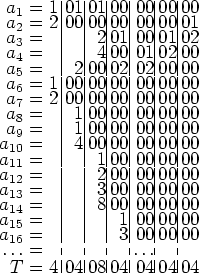The subset-sum problem is shown in Figure 2. For clarity, we have divided the numbers into zones.will be a sum of a subset of theif and only if the totals within each zone are appropriate. Each zone corresponds to one of the logic formulas. For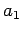through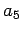, the value in a zone is 0 if the corresponding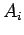does not appear in the logic formula. Ifdoes appear, a power of 2 is used. (the reason for using powers of 2 is that different subsets of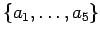will have different totals)

The leftmost zone corresponds to the first logic formula in our example: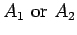. By making suitable decisions about inclusion of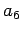or, we will be able to get a total of 4 in this zone, unless bothandare left out of the set, which is precisely what would make the logic formula have value F.

The second zone corresponds to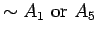, which has value T unlessis in the set andis not.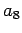,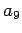, andcan be used to get the total for the zone equal to 4 in any other case.

Similarly, each of the other zones5 hasassociated with it which can be used to obtain the correct total except in one case.

The general problem is that we want numbers which can be used to obtain any total between 1 and, except for one forbidden total''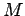. [In the two zones discussed aboveis 4 in one case and 3 in the other] We start with the powers of 2 from 1 to. If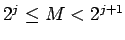, replace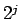by the two numbersand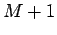. [We did not follow exactly the procedure described in this paragraph in constructing Figure 2.]

#### Footnotes

... zones5
We have omitted thefor the last three zones in Figure 2.Next: Converting (SSP) to (SP) Up: Subset-Sum Problems and NP-Completeness Previous: The Satisfiability Problem   Contents
Translated from LaTeX by Scott Sutherland
2002-12-14